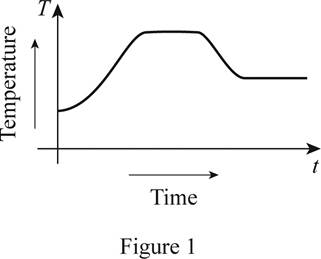# A rough graph of the temperature of the pie as a function of time.### Single Variable Calculus: Concepts...

4th Edition
James Stewart
Publisher: Cengage Learning
ISBN: 9781337687805### Single Variable Calculus: Concepts...

4th Edition
James Stewart
Publisher: Cengage Learning
ISBN: 9781337687805

#### Solutions

Chapter 1.1, Problem 18E
To determine

## To sketch: A rough graph of the temperature of the pie as a function of time.

Expert Solution

### Explanation of Solution

Given:

A frozen pie is placed in an oven and baked for an hour. Then, it is taken out and cooled.

Observation:

Take the time in the x-axis and the temperature in the y-axis. Initially, when the pie is placed in an oven, the temperature of the pie increases and reaches the oven temperature. After it is taken out and cooled, the temperature of the pie decreases to room temperature. Thus, the rough graph of the temperature of the pie as a function of time is shown below in Figure 1.From Figure 1, it is observed that the temperature is steadily increasing at the beginning, remains constant in the middle, then decreases, and stays constant at the end.

### Have a homework question?

Subscribe to bartleby learn! Ask subject matter experts 30 homework questions each month. Plus, you’ll have access to millions of step-by-step textbook answers!